# Lie group, compact

(diff) ← Older revision | Latest revision (diff) | Newer revision → (diff)

A compact group that is a finite-dimensional real Lie group. Compact Lie groups can be characterized as finite-dimensional locally connected compact topological groups.

If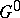is the connected component of the identity of a compact Lie group, then the group of connected componentsis finite. The study of the structure of connected compact Lie groups is a basic topic in the theory of Lie groups.

The following examples of connected compact Lie groups play an important role in the general structure theory of compact Lie groups.

1) The multiplicative group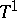of all complex numbers of modulus 1.

2) The group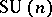of all complex unitary matrices of orderwith determinant 1.

3) The group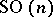of all real orthogonal matrices of orderwith determinant 1.

4) The groupof all matricesfor which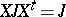, where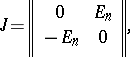is the transposition sign and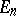is the unit matrix of order.

A complete classification of connected compact Lie groups was obtained in the works of E. Cartan  and H. Weyl . It is as follows.

There are two basic types of connected compact Lie groups.

1) Connected commutative compact Lie groups. These are precisely the tori, that is, groups of the form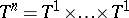(factors) (cf. also Torus).

2) Connected semi-simple compact Lie groups (see Lie group, semi-simple). Ifis a connected semi-simple compact Lie group, then the universal covering groupofis also a compact Lie group (Weyl's theorem). The centre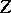of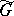is finite, and all connected Lie groups locally isomorphic toare compact and are, up to isomorphism, the groups of the form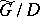, where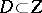. The Lie algebras of semi-simple compact Lie groups can be intrinsically characterized among all finite-dimensional real Lie algebras as algebras with negative-definite Killing form.

The two basic types of connected Lie groups indicated above determine the structure of arbitrary compact Lie groups. Namely, the latter are, up to isomorphism, all groups of the form, whereis a connected simply-connected compact Lie group with centre,is a torus andis a finite subgroup of the groupwhich intersectsonly in the identity. The Lie algebras of arbitrary compact Lie groups can also be characterized intrinsically among all finite-dimensional real Lie algebras: they are precisely the Lie algebrasthat have a positive-definite scalar product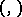such that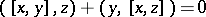for any. They are called compact Lie algebras.

Thus, the classification of connected compact Lie groups reduces to the classification of connected simply-connected semi-simple compact Lie groups (or, equivalently, to the classification of semi-simple compact Lie algebras) and a description of their centres. It turns out that semi-simple compact Lie algebras are in one-to-one correspondence with semi-simple complex Lie algebras (and therefore with reduced root systems, cf. Root system). Namely, ifis a semi-simple compact Lie algebra, then its complexification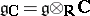is semi-simple. Conversely, in any semi-simple Lie algebra overthere is a unique (up to conjugacy) compact real form (cf. Form of an (algebraic) structure). In particular, the final result on the classification of simple compact Lie algebras and the connected simply-connected compact Lie groups corresponding to them is the following. There are four infinite series of so-called classical simple compact Lie algebras that correspond to the following series of irreducible reduced root systems: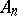,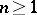,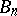,,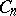,, and,. They are, respectively, the Lie algebras of the groups,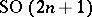,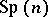, and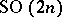. Apart from these there are the five so-called exceptional simple compact Lie algebras corresponding to the root systems of the types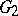,,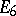,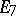, and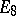. Any compact simple Lie algebra is isomorphic to one of these Lie algebras, and they themselves are not pairwise isomorphic to one another. The compact Lie groupsand,, are connected and simply connected. The group,, is connected but not simply connected. Its universal covering is called the spinor compact Lie group and is denoted by. The centre of a connected simply-connected semi-simple compact Lie group coincides with the centre of the corresponding simply-connected complex Lie group (see Lie group, semi-simple).

Any compact Lie group has a faithful linear representation; the image of such a representation is a real algebraic group. Any compact Lie grouphas a complexification(see Complexification of a Lie group). Moreover,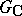is a complex reductive algebraic group (cf. Reductive group) whose affine algebra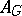can be described as the algebra of all representation functions on, that is, continuous complex-valued functionssuch that the linear envelope of the translates ofby elements ofis finite-dimensional. The algebrahas a natural real structure and so it determines an algebraic group over. The real points of this group form, and the complex points form. The groupis a maximal compact subgroup in. As a result one obtains a one-to-one correspondence between the classes of isomorphic compact Lie groups and reductive algebraic groups over.

Any compact Lie group is a real-analytic group. Compact complex-analytic groups are also called complex compact Lie groups. Any connected complex compact Lie group (as a complex Lie group) is isomorphic to a complex torus, whereis a discrete subgroup of rank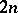in, and (as a real Lie group) it is isomorphic to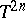. Two complex tori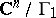and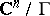are isomorphic (as complex Lie groups) if and only if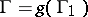for some.

How to Cite This Entry:
Lie group, compact. Encyclopedia of Mathematics. URL: http://encyclopediaofmath.org/index.php?title=Lie_group,_compact&oldid=14523
This article was adapted from an original article by V.L. Popov (originator), which appeared in Encyclopedia of Mathematics - ISBN 1402006098. See original article